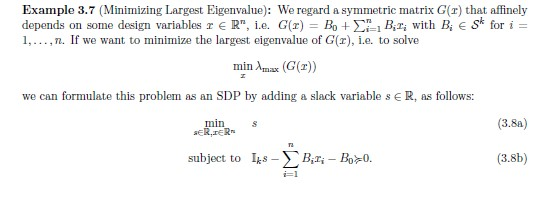# Semidefinite programminghow to write this in cvx?

1 Like

See my answer at Eigenvalue in cvx , and then put in the residual effort to handle this question.

1 Like

but i want to find x where G(X) is minimize

1 Like

`variable x(n)`

then form `G`

`minimize(lambda_max(G))`

That will constrain G to be psd.

1 Like

can you help me in writting G(x)?

1 Like

You need to form the matrix G(x) in CVX the same way you would if x were a MATLAB double precision vector. That is a matter of MATLAB syntax, not anything unique to CVX.

1 Like